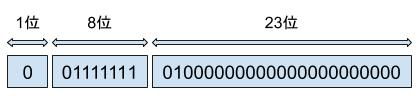### 为什么浮点精度运算会有问题

``````double num = 0.1 + 0.1 + 0.1;
// 输出结果为 0.30000000000000004
double num2 = 0.65 - 0.6;
// 输出结果为 0.05000000000000004``````

``````// 二进制 10010 到十进制
// 从右向左按权相加
10010 = 0 * 2^0
+ 1 * 2^1
+ 0 * 2^2
+ 0 * 2^3
+ 1 * 2^4 = 18

// 十进制 18 到二进制
18 / 2 = 9 .... 0
9 / 2 = 4 .... 1
4 / 2 = 2 .... 0
2 / 2 = 1 .... 0
1 / 2 = 0 .... 1

10010``````
PS：2^3 代表 2 的三次方，以此类推。

``````// 二进制 10.01 到十进制的转化
// 从右向左按权相加，小数点后面的从 -1 开始
10.01 = 1 * 2^-2
+ 0 * 2^-1
+ 0 * 2^0
+ 1 * 2^1 = 2.25

// 十进制 2.25 到二进制的转化
// 因为小数和整数计算方法不一样，这里我们需要把小数和整数分开算。
// 整数部分除 2 ，算出的结果继续除 2 ，直至结果为零结束，每一步计算的余数逆序排列
2 / 2 = 1 .... 0
1 / 2 = 0 .... 1

// 小数部分乘 2 ，算出结果的小数部分继续乘 2 ，直至没有小数为止，每一步计算结果的整数部分顺序排列
0.25 * 2 = 0.5 整数部分为 0
0.5 * 2 = 1 整数部分为 1，后面没有小数，计算结束。

// 结果 10.01``````

``````2.1 分成两部分
// 整数部分
2 / 2 = 1 .... 0
1 / 2 = 0 .... 1

// 小数部分
0.1 * 2 = 0.2 整数部分为 0
0.2 * 2 = 0.4 整数部分为 0
0.4 * 2 = 0.8 整数部分为 0
0.8 * 2 = 1.6 整数部分为 1
0.6 * 2 = 1.2 整数部分为 1
0.2 * 2 = 0.4 整数部分为 0
0.4 * 2 = 0.8 整数部分为 0
0.8 * 2 = 1.6 整数部分为 1
0.6 * 2 = 1.2 整数部分为 1
0.2 * 2 = 0.4 整数部分为 0
0.4 * 2 = 0.8 整数部分为 0
0.8 * 2 = 1.6 整数部分为 1
0.6 * 2 = 1.2 整数部分为 1
..... 小数一直不为 0 一直计算下去 .......``````

### 精度运算丢失的解决办法

1. 如果业务不是必须非常精确的要求可以采取四舍五入的方法来忽略这个问题。
2. 转成整型再进行计算。
3. 使用 BCD 码存储和运算二进制小数(感兴趣的同学可自行搜索学习)。

``````# Python 示例
from decimal import Decimal

num = Decimal('0.1') + Decimal('0.1') + Decimal('0.1')
print(num)``````
``````// Java 示例
import java.math.BigDecimal;

### 拓展：详解浮点型0 是正数，1 是负数

##### 尾数位

``````import struct

num = 1.1
bins = ''.join('{:0>8b}'.format(c) for c in struct.pack('!f', num))
print(bins)``````
Java 版本的代码有点长，我就放个链接了。
Java 版代码链接